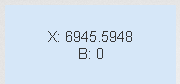# QlikView Creating Analytics

Discussion Board for collaboration related to Creating Analytics for QlikView.Not applicable

## Linear regression Linest_M + Linest_B

Hi all,

I want to forecast the amount of orders for the coming months. I've created a master calendar which adds dates for + 1 year after the max date in the data (so that I can forecast multiple months ahead).

However, the linest_m and linest_b aren't calculating the correct numbers.

To calculate the forecast I'm using the following functions:

(LINEST_M( TOTAL Aggr(Count([Werkorder partner]), [Maand melding]), [Maand melding]) * NUM([Maand melding]) )+

LINEST_B(TOTAL Aggr(Count([Werkorder partner]), [Maand melding]), [Maand melding])

As you can see the X is 72,30, when I calculate it manually however I get: 903 / 8 = 112,875 and B -111,875.

I've tried forcing the graph to start in (0,0) with

(LINEST_M( TOTAL Aggr(Count([Werkorder partner]), [Maand melding]), [Maand melding],0,0)

but this returns x as 6945,5948???The correct formula (I think) should be: 112,875X - 111,875. Just have to get qlik to understand that1 Solution

Accepted SolutionsMVP

## Re: Linear regression Linest_M + Linest_B

7 RepliesMVP & Luminary

## Re: Linear regression Linest_M + Linest_B

The chart doesn't start at 0. It starts at Jan. That's the first 'row' of the chart.

talk is cheap, supply exceeds demandMVP

## Re: Linear regression Linest_M + Linest_BNot applicable

## Re: Linear regression Linest_M + Linest_B

So you suggest I should use linest_m( ....,..., 0,1)? I'll test it tonight.Not applicable

## Re: Linear regression Linest_M + Linest_B

I'm not sure if I understand the post, you suggest to rank the month dimension in the linest_ functions?Not applicable

## Re: Linear regression Linest_M + Linest_B

Converting the dimension doesn't fixed the problem.HighlightedMVP

## Re: Linear regression Linest_M + Linest_B

Could you upload a small sample QVW?Not applicable

## Re: Linear regression Linest_M + Linest_B

Okay got the linear regression correct, as in equal to the one qlikview calculates. But I still think the regression should be 112,875X - 111,875 not 72,30x + 150,44 as qlikview calculates. I think this has to do with the fact that a count of the orders in january returns null, as well as okt nov dec.